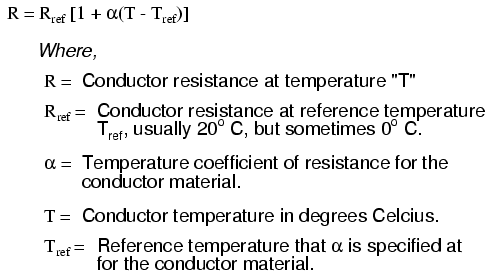The Temperature Coefficient of Copper (near room temperature) is +0.393 percent per degree C. This means if the temperature increases 1°C, the resistance will increase 0.393%.

Examples:

You have 100 feet of 20 gauge wire and its resistance is 1.015 ohms at 20° C (room temp). If the temperature of the wire goes up 10°C, the resistance will change by 0.0399 ohms (10 degrees * 0.00393 per degree * 1.015 ohms = 0.0399 ohms).

The wire resistance will now be 1.015 ohms + 0.0399 ohms = 1.0549 ohms.

You have 1 foot of ribbon cable with a resistance of 0.0649 ohms at 20 degrees C. You plug the wire into your cable tester and keep your hands on the wire while it tests. The wire temperature goes up 10°C because of your body heat. The wire resistance will go up 0.00255 ohms (10 degrees * 0.00393 per degree * 0.0649 ohms = 0.00255 ohms).

While the wire resistance changes about 4%, the total change is only 2.6 milliohms--which is a very minor change.

You can find more details about temperature coefficients of resistance here and a calculator here.

The generic forumla for temperature affects on resistance is as follows:The temperature coefficient for some common materials are listed below (@ 20ºC):

• Copper = 0.00393
• Aluminum = 0.004308
• Iron = 0.005671
• Nickel = 0.005866
• Gold = 0.003715
• Tungsten = 0.004403
• Silver = 0.003819